## 从零开始用 Python 构建神经网络

动机：为了更加深入的理解深度学习，我们将使用 python 语言从头搭建一个神经网络，而不是使用像 Tensorflow 那样的封装好的框架。我认为理解神经网络的内部工作原理，对数据科学家来说至关重要。

• 一个输入层，x
• 任意数量的隐藏层
• 一个输出层，ŷ
• 每层之间有一组权值和偏置，W and b
• 为隐藏层选择一种激活函数，σ。在教程中我们使用 Sigmoid 激活函数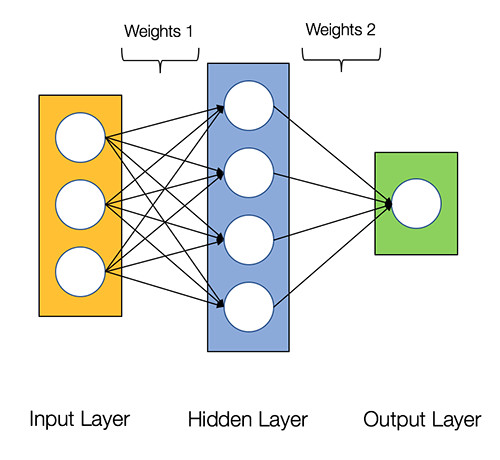2 层神经网络的结构

class NeuralNetwork:

def __init__(self, x, y):

self.input = x

self.weights1 = np.random.rand(self.input.shape,4)

self.weights2 = np.random.rand(4,1)

self.y = y

self.output = np.zeros(y.shape)

• 计算预测结果 ŷ，这一步称为前向传播
• 更新 W 和 b,，这一步成为反向传播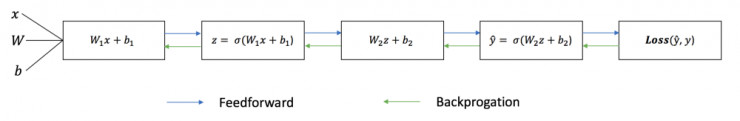class NeuralNetwork:

def __init__(self, x, y):

self.input = x

self.weights1 = np.random.rand(self.input.shape,4)

self.weights2 = np.random.rand(4,1)

self.y = y

self.output = np.zeros(self.y.shape)

def feedforward(self):

self.layer1 = sigmoid(np.dot(self.input, self.weights1))

self.output = sigmoid(np.dot(self.layer1, self.weights2))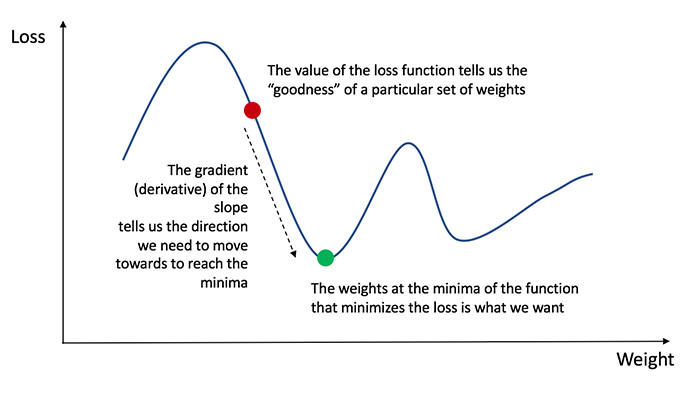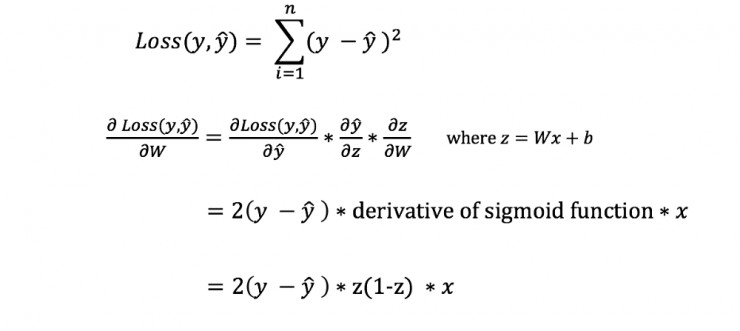class NeuralNetwork:

def __init__(self, x, y):

self.input = x

self.weights1 = np.random.rand(self.input.shape,4)

self.weights2 = np.random.rand(4,1)

self.y = y

self.output = np.zeros(self.y.shape)

def feedforward(self):

self.layer1 = sigmoid(np.dot(self.input, self.weights1))

self.output = sigmoid(np.dot(self.layer1, self.weights2))

def backprop(self):

# application of the chain rule to find derivative of the loss function with respect to weights2 and weights1

d_weights2 = np.dot(self.layer1.T, (2*(self.y - self.output) * sigmoid_derivative(self.output)))

d_weights1 = np.dot(self.input.T, (np.dot(2*(self.y - self.output) * sigmoid_derivative(self.output), self.weights2.T) * sigmoid_derivative(self.layer1)))

# update the weights with the derivative (slope) of the loss function

self.weights1 += d_weights1

self.weights2 += d_weights2

Youtube：https://youtu.be/tIeHLnjs5U8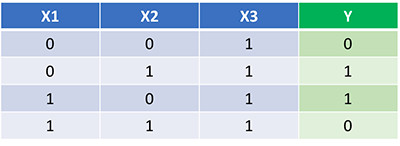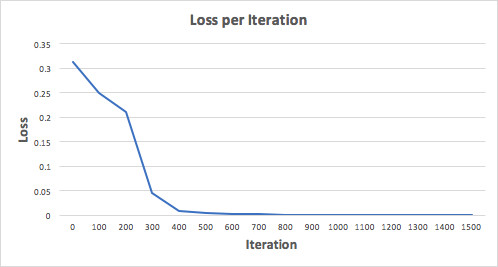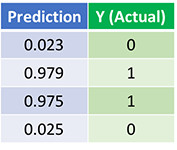• 除了 Sigmoid 以外，还可以用哪些激活函数
• 在训练网络的时候应用学习率
• 在面对图像分类任务的时候使用卷积神经网络